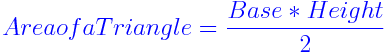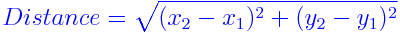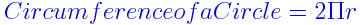# Measurement Formulas

Measurements are used to measure something. It is the estimation of ratios of quantity. It is made by comparing a quantity with a standard unit. They are used to find the size, length or amount of something. Measurement Formulas are used to find the distance, area, surface area, volume, circumference etc. They also include some conversion formulas like conversion of an inch to feet, meter to miles etc.

Some of the Measurement Formulas are given below:## Measurement Problems

Some solved problems based on measurements are given below:

### Solved Examples

Question 1: Find the area of a triangle with base 3 units and height 8 units?
Solution:

Base = 3 units
Height = 8 units
Area of a Triangle=
$$\begin{array}{l}frac{base*height}{2}\end{array}$$
=
$$\begin{array}{l}frac{3 \times 8}{2}\end{array}$$

=

$$\begin{array}{l}frac{24}{2}\end{array}$$

= 12 sq.units

Question 2: Find the distance between two points (2, 3) and (7, 5)?
Solution:

(x1, y1) = (2, 3)
(x2, y2) = (7, 5)
Distance between the points=
$$\begin{array}{l}sqrt{(x_{2} – x_{1})^{2} + (y_{2} – y_{1})^{2}}\end{array}$$
=
$$\begin{array}{l}sqrt{(7 – 2)^{2} + (5 – 3)^{2}}\end{array}$$

=
$$\begin{array}{l}sqrt{29}\end{array}$$

= 5.39 units

 More topics in Measurement Formulas Square Footage Formula Temperature Conversion Formula Unit Rate Formula Degree and Radian Measure Formula

Measurements are used to measure something. It is the estimation of ratios of quantity. It is made by comparing a quantity with a standard unit. They are used to find the size, length or amount of something. Measurement Formulas are used to find the distance, area, surface area, volume, circumference etc. They also include some conversion formulas like conversion of an inch to feet, meter to miles etc.

Some of the Measurement Formulas are given below:## Measurement Problems

Some solved problems based on measurements are given below:

### Solved Examples

Question 1: Find the area of a triangle with base 3 units and height 8 units?
Solution:

Base = 3 units
Height = 8 units
Area of a Triangle=
$$\begin{array}{l}\frac{base*height}{2}\end{array}$$
=
$$\begin{array}{l}\frac{3 \times 8}{2}\end{array}$$

=

$$\begin{array}{l}\frac{24}{2}\end{array}$$

= 12 sq.units

Question 2: Find the distance between two points (2, 3) and (7, 5)?
Solution:

(x1, y1) = (2, 3)
(x2, y2) = (7, 5)
Distance between the points=
$$\begin{array}{l}\sqrt{(x_{2} – x_{1})^{2} + (y_{2} – y_{1})^{2}}\end{array}$$
=
$$\begin{array}{l}\sqrt{(7 – 2)^{2} + (5 – 3)^{2}}\end{array}$$

=
$$\begin{array}{l}\sqrt{29}\end{array}$$

= 5.39 units

 More topics in Measurement Formulas Square Footage Formula Temperature Conversion Formula Unit Rate Formula Degree and Radian Measure Formula